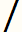# Egyptian Fractions Calculator

Ancient Egyptians had a peculiar way of expressing fractions. For example, rather than write the quantity three fourths as 3/4, they preferred to express the quantity as the sum of distinct unit fractions (fractions with a numerator of 1). Thus, 3/4 was written as 1/2 + 1/4.

Every rational number between 0 and 1 has an Egyptian fraction representation, that is, it can be written as a finite sum of unit fractions. The representation is not unique. For example, the fraction 5/16 can be written as:
 1/4 + 1/16 1/5 + 1/9 + 1/720 1/5 + 1/10 + 1/80 1/5 + 1/12 + 1/35 + 1/1680 1/5 + 1/13 + 1/29 + 1/914 + 1/13783120 1/6 + 1/7 + 1/336 1/6 + 1/8 + 1/48 1/6 + 1/9 + 1/29 + 1/4176 1/6 + 1/12 + 1/16 1/7 + 1/8 + 1/28 + 1/112

### Egyptian Fraction Expansion Calculator### Greedy Algorithm For Egyptian Fractions

The simplest method for expressing a vulgar fraction as the sum of unit fractions is to use a greedy algorithm. In this method, you subtract the largest possible unit fraction from the given fraction, and then continue by subtracting the largest possible unit fraction from the remainder at each step. The greedy algorithm always terminates and the maximum number of summands required is equal to the value of the numerator. Usually the algorithm terminates much sooner than that.

While the greedy algorithm always produces an answer, it does not guarantee the most compact or "simplest" unit fraction expression. For example, applying the greedy Egyptian fraction algorithm to 5/121 yields

1/25 + 1/757 + 1/763309 + 1/873960180913 + 1/1527612795642093418846225

However, there are several much simpler expressions for 5/121:

1/26 + 1/350 + 1/275275
1/27 + 1/234 + 1/84942
1/33 + 1/91 + 1/33033
1/33 + 1/93 + 1/3751
1/33 + 1/99 + 1/1089
1/33 + 1/121 + 1/363
1/34 + 1/84 + 1/172788
1/44 + 1/55 + 1/2420

### Other Methods of Finding Egyptian Fractions

Given a reduced fraction x/y and a particular number n, consider the fraction (xn)/(yn). If you can express xn as the sum of some distinct divisors of yn, then the fraction will easily split into unit fractions.

For example, take the fraction 19/289 = 19/17². Using the greedy algorithm yields the unwieldy expansion

1/16 + 1/309 + 1/129893 + 1/26513313813 + 1/1640230221782258750448.

However, using n = 5*29 = 145, we can consider instead the equivalent fraction 2755/41905. Notice that

2755 = 2465 + 289 + 1

and that 2465, 289, and 1 are all divisors of 41905. Thus, 2755/41905 can be written as

(2465 + 289 + 1)/41905 = 1/17 + 1/145 + 1/41905

which yields a much simpler Egyptian fraction representation of 19/289. Of course, finding the magic value of n is not trivial.